## Friday, August 29, 2014

### Why sin^2 x + cos^2 x = 1

Why does sin^2 x + cos^2 x = 1?  Find out in this video.

- Eddie

### Moore-Penrose Inverse of a Matrix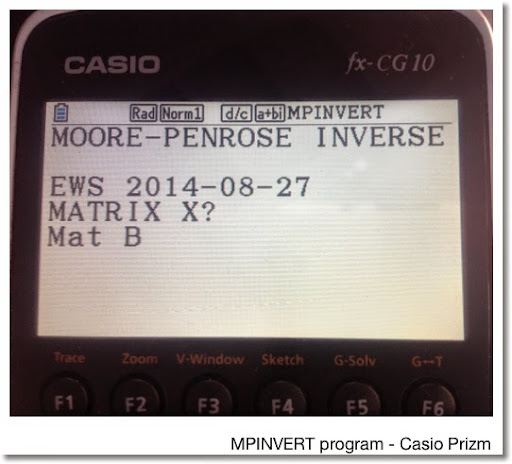Moore-Penrose Inverse of a Matrix
(E.H. Moore (1920), Arne Bjerhammar and Roger Penrose (1955))

Programs for the HP Prime and Casio Prizm (can be used for fx-9860g II and fx-9750g II) included

Also known as a pseudoinverse, the Moore-Penrose inverse of a matrix, denoted by A^+ (capital A with a supersubscript of a plus sign), is an inverse of matrix. Different from the "true" matrix inverse, the Moore-Penrose inverse allows for non-square matrices. Primarily, the Moore-Penrose inverses are calculated is assist in solving linear least-square equations.

Properties: Moore-Penrose Inverse

A A^+ A = A
A^+ A A^+ = A^+
(A A^+)^T = A
(A^+ A)^T = A^+

A^+ is unique

Calculation

One method of calculating the Moore-Penrose Inverse is the following:

For matrix A of size m × n:

Let r = row rank of A (number of linearly independent rows)
Let c = column rank of A (number of linearly independent columns)
Usually r = c
The matrix rank, denoted rank(A) = min(r, c)

If r ≤ c and rank(A) = r,
Then A^+ = A^T (A A^T)^-1

If r ≥ c and rank(A) = c,
Then A^+ = (A^T A)^-1 A^T

The programs listed on this blog entry assume the first conditions of each of the test are met, since usually, r = c.

Sources

Schott, James R. "Matrix Analysis for Statistics: 2nd Edition". Wiley & Sons. Hoboken, NJ. 2005

Wikipedia article, retrieved 8/29/2014 - URL:
http://en.m.wikipedia.org/wiki/Moore–Penrose_pseudoinverse

HP Prime Program MPINVERT

Input: MPINVERT(matrix). Execute this from Home or CAS screen.

Program:
EXPORT MPINVERT(mtx)
BEGIN
// 2014-08-27 EWS
// Moore-Penrose Matrix Inverse
LOCAL r,c,d,n;
d:=SIZE(mtx);
r:=d(1);
c:=d(2);
n:=RANK(mtx);
CASE
IF n==c THEN
RETURN (TRN(mtx)*mtx)^-1*mtx;
END;
IF n==r THEN
RETURN TRN(mtx)*(mtx*TRN(mtx))^-1;
END;
DEFAULT
RETURN "No Solution Found";
END;

END;

Casio Prizm Program MPINVERT

The program prompts for the matrix. Since the Prizm does not have a matrix rank command, it is determined by use of the rref command and pair of For loops. This program should work verbatim on the fx-9860g II and fx-9750g II.

The matrix is stored in Mat X. List 1 and Mat Y are used for calculation. The result is stored in Mat Z.

If you are using a TI-84, you would probably use an approach similar to what is presented:

Program MPINVERT
"MOORE-PENROSE INVERSE"
"EWS 2014-08-27"
"MATRIX X"? → Mat X
Dim Mat X→List 1
List 1 → R
List 1 → C
Rref Mat X → Mat Y
0 → T
For 1 → I To R
For 1 → J To C
If Mat Y[I, J] ≠ 0
Then
T + 1 → T
Break
IfEnd
Next
Next
If T = R
Then
Trn Mat X * (Mat X * Trn Mat X) ^ -1 → Mat Z
"Mat Z"
Mat Z ◢ // display
Stop
IfEnd
If T = C
Then
(Trn Mat X * Mat X) ^ -1 * Trn Mat X → Mat Z
"Mat Z"
Mat Z ◢ // display
Stop
IfEnd
"NO INVERSE"

Examples

Matrix:
[ [ 1, 2, 3 ] [ 3, 4, 0 ] ]

Moore-Penrose Inverse:
[ [ -8/229, 31/229 ] [ 6/229, 34/229 ] [ 75/229, -33/229 ] ]

Matrix:
[ [7, 4, 6, -7] [-1, 5, 3, 3] ]

Moore-Penrose Inverse: (to four decimal places)
[ [0.0489, -0.0338] [0.0194, 0.1092] [0.0360, 0.0600] [-0.0520, 0.0800] ]

This blog is property of Edward Shore. 2014

## Saturday, August 23, 2014

### General Calculator Advice for School (and Possibly Beyond)

General Calculator Advice for School (and Possibly Beyond)

Last week, I received several emails that ask me to make recommendations for which calculators to purchase. Today I will outline my general thoughts about calculators.

Note: I will assume that tablets, such as the Microsoft Surface and Apple iPad are not allowed in classroom tests. Therefore, the focus of this blog entry will be on physical, tangible calculators and not calculator apps found on tablets and phones.

Non-Graphing Calculators

Today's non-graphing scientific calculators are packed with features. The high-end models include:

* numerical derivatives and numerical integration calculations
* textbook input and output, which includes fractions, exact expressions of π, and exact roots
* a general root finder, along with solvers for two simultaneous equations, three simultaneous equations, quadratic equations, and cubic equations
* statistics with regression analysis
* basic matrix operations (determinant, inverse)
* table generator of a function f(x)
* decimal, binary, hexadecimal, and octal conversions
* a library of conversions and constants (all vary)

If you are interested in obtaining a non-graphing calculator and aim to get the most for your money, I recommend any of the following models. Links of my review are listed below:

Texas Instruments TI-36X Pro
http://edspi31415.blogspot.com/2011/04/ti-36-pro-review.html

Casio fx-115ES Plus
http://edspi31415.blogspot.com/2012/04/review-casio-fx-115-es-plus-review.html

Cannon F-792SGA
http://edspi31415.blogspot.com/2014/05/review-canon-f-792sga-scientific.html

Sharp EL-W516X
http://edspi31415.blogspot.com/2011/09/sharp-el-w516x-review.html

All are solar powered (partially) and that extends the life of the battery. Each can be obtained for approximately \$16 - \$20, although some office stores do sell the TI-36X as high as \$25. The differences between each of the calculators are fairly minor. The interface of the TI-36X has more integrated interface than the others, that is, you will not need to go into separate modes to use matrices and complex numbers. Other than that, any of the four listed above is a solid choice.

The non-graphing calculator that is not solar is the HP 35s from Hewlett Packard. What separates the HP 35s from the others listed is:

* The HP 35s is programmable. Each program is a list of keystrokes. The 35s has about 32,000 bytes of memory.
* The 35s operates in both algebraic and RPN (Reverse Polish Notation) modes. The algebraic mode is not textbook entry.
* Complex number support includes powers, trigonometric functions, exponential functions, and logarithmic functions.
* The factorial function (x!) accepts any real number, not just positive integers.
* The 35s runs on batteries (two CR 2032 batteries)
* The 35s is typically priced in the \$50 - \$60 range.

Solvers on the 35s include simulteanous equations of order 2 and 3, and a general root finder. Numerical integrals are included.

Graphing Calculators

Most students will probably use a graphing calculator sometime during their studies. Here are some of my thoughts:

TI-83 Plus vs TI-84 Plus: I lean towards the TI-84 Plus simply because it has a faster processor, the display is crisper, and the TI-84 Plus offers textbook entry and output mode (known as MathPrint).

Programming wise, they have 24,000 bytes of RAM. Fortunately, they have a lot more of flash ROM memory which acts like archived storage. (512,000 bytes for the 83 Plus vs 1 MB for the 84 Plus). The archived storage contains programs and calculator applications.

Retail wise, the difference in price is about \$10. The 83 Plus costs about \$90 retail versus \$100 for the 84 Plus.

TI-84 Plus vs TI-84 Plus C Silver Edition: A question of color. Back to school time is a best time to find the TI-84 Plus C Silver Edition at its best price, which normally is about \$120 to \$140.

My review of the color edition is here:
http://edspi31415.blogspot.com/2013/04/ti-84-plus-c-silver-edition-review.html

Basically, while the color and the rechargeable battery are good additional features, processing sometimes suffers. Other not so good points about the TI-84 C Silver Edition is that the graphing screen is smaller, and we only have 21,000 bytes of RAM to work with.

Of these two, I have to give the edge to the older, monochrome displayed TI 84 Plus.

I personally would not recommend the TI nSpire CX unless you get the CAS edition. I will talk about calculators with CAS in the next edition.

Casio may be cheaper, but is it better? I believe the Casio calculators can go toe to toe with the Texas Instruments counterparts. The user interface is comparable if not easier, and you can mix graph types (function, parametric, and polar) on one graphing screen. All three models I am about to describe have 62,000 bytes of programmable memory and uses a menu and soft key interface. Basically, if you can operate one, you should be easily be able to handle the others.

No worries about whether the teacher can operate a Casio, I recently have seen the Casio models fly off the shelves (at least in the eastern Los Angeles area).

fx-9750G II: This is the basic model, priced around \$50. All input and output is algebraic (no textbook input or output). Otherwise, it may be something to consider if you don't want to spend much money or not a fan of math and just need a graphing calculator to get by. I don't have an fx-9750G II - yet and interested in adding this to my collection.

fx-9860G II: This has all the features of the fx-9750G II plus a geometry app, spreadsheet app, textbook input and output (MathIO), backlit screen, and Flash ROM memory. Price typically range from \$75 to \$90.

fx-CG 10 (fx-CG 20 outside of the United States), better known as the Prizm: This is the fx-9860G II but with color and higher Flash ROM memory. The screen on the Prizm is awesome. Priced about \$100.

Prizm vs TI-84 Plus C Silver Edition: Prizm is my choice, hands down.

My choices for recommendations would be: TI-84 Plus (classic monochrome), Casio Prizm.

If you are on a budget and textbook input and output is not a must have, you may want to consider the Casio fx-9750G II.

Calculators with CAS

This class is the upper echelon of calculators, those with a CAS (computer algebraic system). I would only recommend that you consider buying a calculator with CAS if any of the following apply:

* You are in college and plan to obtain a degree in mathematics, engineering, or physics.
* You are planning to take multi-variable calculus, differential equations, and any math classes beyond this level.
* You are a mathematics enthusiast.

Models to consider are these:

Hewlett Packard: HP 50g. I would consider this the standard when it comes to calculators with a CAS. Features include an equation writer, RPN mode, matrix entry mode, multi variable solver, solver templates for general equations, polynomials, and simultaneous equations, and 3D graphing. The 50g has an SD card slot. RPN mode truly shines with the 50g. The current price is about \$100.

Hewlett Packard: HP Prime: This is HP's color graphing calculator. I think this has the fastest processor of any calcualtor. The memory is 32 MB, which is a ton. The calculator also has a touch screen, which allows for cursor placement and zooming on graphs. It is powered with a rechargeable battery. Check out the Advanced Graphing app where it is easy to execute implicit plots and open statements. (For example x^2 + y^2 <= 4). The downside is that the RPN mode is very limited. The current price is around \$150.

Three other calculators with CAS to consider are:

Texas Instruments: TI nSpire CX CAS: This does not have a touch screen but has a touchpad. I really like the geometry app on this model. Like the Prime, the CX CAS has a color screen and has a rechargeable battery. The user interface is like Microsoft Office, files are created for each project. My biggest gripe about the nSpire is the programming is severely limited and can only interact with home pages. Price is about \$150.

Texas Instruments TI-89 Titanium: I would rank this calculator higher except that the screen kind of sucks. The font is tiny and there is little contrast to the screen. Feature wise, then TI-89 is the most advanced of all Texas Instruments calculators, despite the latest operating system was release in 2005. Price is about \$150.

Casio ClassPad fx-CP400: I don't have the newest ClassPad, but from what I read it is an upgrade to the ClassPad 330. The screen is large, the keyboard is limited, you do mostly everything with a stylus, and the screen rotated. I think the price is about \$150.

My recommendations for calculators with a CAS are the HP 50g and HP Prime. Search my blog for basic programming tutorials for both the HP 50g (RPL Programming Tutorial) and HP Prime.

Hope this helps, and if you have questions, please ask and I will try the best I can to assist. Many thanks for the comments and questions.

Eddie

This blog is property of Edward Shore. 2014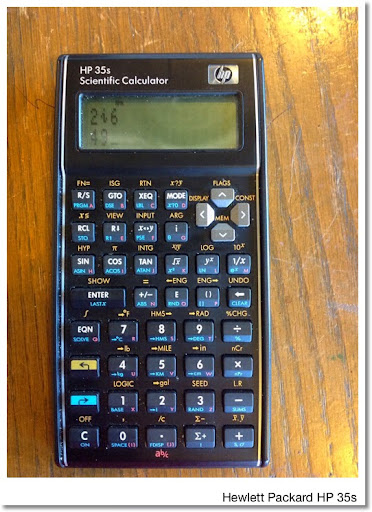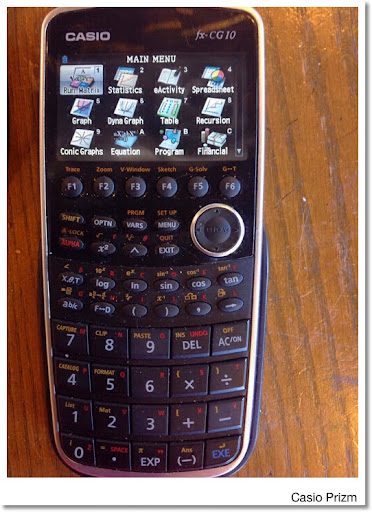## Friday, August 22, 2014

### Ice Bucket Challenge (Overtime)

A little bit of a detour from mathematics...

I have been challenged by my best friend since childhood, Robert, and his family to do the ALS Ice Bucket Challenge.  This is to help raise awareness and funds to help find a cure for ALS, commonly known as Lou Gehrig's Disease.   Cold water is refreshing given how hot the summer days and nights have been!

My video:  http://youtu.be/56UGAtazrwE

Website:  www.alsa.org

I will be making a donation and encourage anybody who wants to participate in the ice bucket challenge and/or (more importantly) make a donation.

Eddie

## Thursday, August 21, 2014

### Pythonista 2.7 and HP 35S: Given roots of a polynomial, find the coefficients of a polynomial

General

Variables:
Number of Roots: N
Roots: R, S, T, U
Coefficients: A, B, C, D, E

N = 2, roots R and S:
(x - R) * (x - S) → A * x^2 + B * x + C

Formulas:
A = 1
B = -(R + S)
C = R * S

N = 3, roots R, S, and T:
(x - R) * (x - S) * (x - T) → A * x^3 + B * x^2 + C * x + D

Formulas:
A = 1
B = -(R + S + T)
C = R * S + R * T + S * T

N = 4, roots R, S, T, and U:
(x - R) * (x - S) * (x - T) * (x - U) → A * x^4 + B * x^3 + C * x^2 + D * x + E

Formulas:
A = 1
B = -(R + S + T + U)
C = R * S + R * T + R * U + S * T + S * U + T * U
D = -(R * S * T + R * S * U + R * T * U + S * T * U)
E = R * S * T * U

HP-35S: Coefficients To Roots

Program:
C001 LBL C
C002 SF 10 // SF, decimal point, 0
C003 NO OF ROOTS // enter message as an equation
C004 CF 10 // CF, decimal point, 0
C005 INPUT N
C006 4 // error checking
C007 xC008 GTO C113
C009 R-down
C010 2
C011 x>y?
C012 GTO C113
C013 1 // main routine
C014 STO A
C015 INPUT R
C016 INPUT S
C017 RCL N
C018 3
C019 x=y?
C020 GTO C033
C021 R-down
C022 4
C023 x=y?
C024 GTO C054
C025 RCL R // two roots
C026 RCL+ S
C027 +/-
C028 STO B
C029 RCL R
C030 RCLx S
C031 STO C
C032 GTO C101
C033 INPUT T // three roots
C034 RCL R
C035 RCL+ S
C036 RCL+ T
C037 +/-
C038 STO B
C039 RCL R
C040 RCLx S
C041 RCL R
C042 RCLx T
C043 +
C044 RCL S
C045 RCLx T
C046 +
C047 STO C
C048 RCL R
C049 RCLx S
C050 RCLx T
C051 +/-
C052 STO D
C053 GTO C101
C054 INPUT T // four roots
C055 INPUT U
C056 +
C057 RCL+ S
C058 RCL+ R
C059 +/-
C060 STO B
C061 RCL R
C062 RCLx S
C063 RCL R
C064 RCLx T
C065 +
C066 RCL R
C067 RCLx U
C068 +
C069 RCL S
C070 RCLx T
C071 +
C072 RCL S
C073 RCLx U
C074 +
C075 RCL T
C076 RCLx U
C077 +
C078 STO C
C079 RCL R
C080 RCLx S
C081 RCLx T
C082 RCL R
C083 RCLx S
C084 RCLx U
C085 +
C086 RCL R
C087 RCLx T
C088 RCLx U
C089 +
C090 RCL S
C091 RCLx T
C092 RCLx U
C093 +
C094 +/-
C095 STO D
C096 RCL R
C097 RCLx S
C098 RCLx T
C099 RCLx U
C100 STO E
C101 VIEW A // results
C102 VIEW B
C103 VIEW C
C104 RCL N
C105 3
C106 x≤y?
C107 VIEW D
C108 RCL N
C109 4
C110 x=y?
C111 VIEW E
C112 RTN
C113 0 // invoking the error condition
C114 1/x

Pythonista

Input: Enter a vector of coefficients, up to 4 roots
Output: A list of coefficients, in descending order

Note: the triple periods indicate a tab (...)

# let roots be the list of roots, up to 4
# EWS 2014-08-20
import math
roots=input('List of Roots (up to 4):')
n=len(roots)
poly=
# check for order
if n==2:
...poly.append(-(roots+roots))
...poly.append(roots*roots)
...print('List of coefficients: ',poly)
elif n==3:
...# cubic
...temp=-(roots+roots+roots)
...poly.append(temp)
...temp=roots*roots+roots*roots+roots*roots
...poly.append(temp)
...temp=-roots*roots*roots
...poly.append(temp)
...print('List of coefficients: ',poly)
elif n==4:
...# quartic
...temp=-(roots+roots+roots+roots)
...poly.append(temp)
...temp=roots*roots+roots*roots+roots*roots+roots*roots+roots*roots+roots*roots
...poly.append(temp)
...temp=-(roots*roots*roots+roots*roots*roots+roots*roots*roots+roots*roots*roots)
...poly.append(temp)
...temp=roots*roots*roots*roots
...poly.append(temp)
...print('List of Coefficients: ',poly)
else:
...print('Error: not a valid list')

Examples:

R = 2, S = -4
A = 1, B = 2, C = -8

Cubic:
R = 3, S = -1, T = -3
A = 1, B = 1, C = -9, D = -9

Quartic:
R = 3, S = -1, T = -3, U = 4
A = 1, B = -3, C = -13, D = 27, E = 36

Eddie

This blog is property of Edward Shore. 2014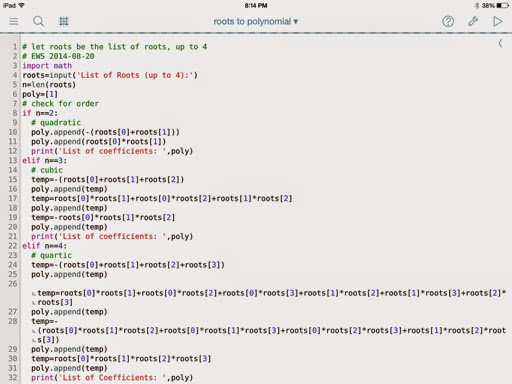## Saturday, August 16, 2014

### Pythonista 2.7: List of Primes, Rotating a List, Maximum of a List, Vandermonde Matrix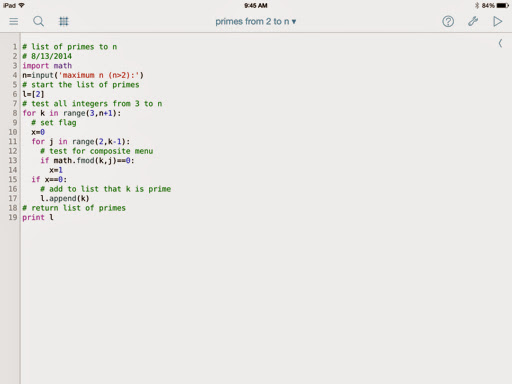List of Primes

# list of primes to n
# 8/13/2014
import math
n=input('maximum n (n>2):')
# start the list of primes
l=
# test all integers from 3 to n
for k in range(3,n+1):
# set flag
x=0
for j in range(2,k-1):
if math.fmod(k,j)==0:
x=1
if x==0:
# add to list that k is prime
l.append(k)
# return list of primes
print l

Example: n = 44 returns

[2, 3, 5, 7, 11, 13, 17, 19, 23, 29, 31, 41, 43]

I am getting used to the way the range command operates and how the indices go from 0 to n-1, instead of how programming calculators have it: 0 to n.

Rotate a List to the Right

# rotate a list to the right r places
import math
l=input('list: ')
r=input('number of places: ')
n=len(l)
# rotation loop
for k in range(r):
# remember range starts at 0
# remove last element
w=l.pop()
# insert that element at position 0
l.insert(0,w)
# print rotated list
print l

Example:

List: [0, 4, -6, 8]

Number of Places: 2; return [-6, 8, 0, 4]
Number of Places: 3; return [4, -6, 8, 0]

Maximum of a List

import math
l=input('list: ')
# sort the list - without a loop
l.sort()
print l.pop()

Example: [12, 16, 28, 3, 4] returns 28

Vamdermonde Matrix

# building the Vandermonde matrix a row at a time
import math
v=input('vector: ')
n=len(v)

print('Vandermonde Matrix')

# main routine
# power
for i in range(n):
l=[]
# element build
for k in range(n):
l.append(math.pow(v[k],i))
print l

Example:
[0.8, 0.6, -0.5] returns

[1, 0.8, 0.64]
[1, 0.6, 0.36]
[1, -0.5, 0.25]

Enjoy!

Have a great weekend!

Eddie

This blog is property of Edward Shore. 2014## Saturday, August 9, 2014

### HP RPN Advance Functions Video Up and Running

This is part two of the RPN Mode for HP Prime tutorial.  This time I am taking about advanced functions, such as numeric integrals, numeric derivatives, summation, and solving equations.

http://youtu.be/YAJwjpldiak

DETAILS:

Syntax - RPN Mode:
-----
MAX and MIN
Path:  Toolbox, Math, 2, 1 for MAX (2 for MIN)

Syntax:
1:  list or vector
MAX(1) or MIN(1)
- or -
Numbers on the stack
MAX(n) or MIN(n)   (n is 9 or less)
-----
Factorizing Integers – CAS.ifactor

1.  Enter the integer to be factorized, press the Enter key.
2.  Press Toolbox, CAS, 5, 2, 1 to execute CAS.ifactor(1)
-----
Generating a Sequence - MAKELIST
Path:  Toolbox, Math, 6, 1

Stack:
5:  ‘function in single quotes’
4:  ‘variable in single quotes’
3:  beginning point
2:  ending point
1:  increment/decrement
MAKELIST(5)
-----
Finding the Roots of a Polynomial - CAS. proot
Path:  Toolbox, CAS, 6, 1

Stack:
1:  vector or list of coefficients (decreasing power of x)
CAS.proot(1)
-----
Summation – CAS.sum
Path: Toolbox, Math, 2, 5

Stack:
4:  ‘function in single quotes’
3:  ‘variable’
2:  starting point
1:  finishing point
CAS.sum(4)
-----
Numerical Integration
1.  Press the Template Key, choose ∫ (2nd row, 4th column)
2.  Fill in the template – use capital letters for the variable
3.  Press the Enter Key
4.  Finally, press Toolbox, CAS, 1, 1 to execute CAS.simplify(1)
-----
Numerical Derivative
1.  Store value in the variable: Number, Enter Key, Variable in single Quotes, Shift Key, EEX Key
2.  Press the Template Key, choose ∂ (1st row, 4th column).  Fill in the template – use capital letters
for the variable, and press the Enter Key
3.  Finally, press Toolbox, CAS, 1, 1 to execute CAS.simplify(1)
-----
Matrix Operations
Put the matrix on the stack.  Use the Template Key, choose matrix (1st row, 6th column).

Determinant:  Press Toolbox, Math, 7, 2.  Determinant is calculated automatically.
Inverse:  Press Shift, then the division key. (x^-1).  This works for square matrices only.
Eigenvalues:  Press Toolbox, Math, 7, 6, 1.  CAS.EIGENVAL is called.  Execute CAS.EIGENVAL(1).
-----
Finding Numerical Roots using FNROOT
Path:  Toolbox, Math, 2, 4
Stack:
3:  ‘function in single quotes’
2:  ‘variable’
1:  guess
Execute FNROOT(3)

Format:   function = 0
-----

Eddie

This blog is property of Edward Shore.  2014

## Thursday, August 7, 2014

### HP Prime Tutorial Videos

There are three new videos up on my YouTube page, which all are tutorials for the HP Prime:

Calculating the Signed Area (Integral):
http://youtu.be/HeX_jLB5s1w

Using the Solve App:
http://youtu.be/PVZNP1NMhvk

RPN Mode Basics:
http://youtu.be/_eHXyGm9sEE

Eddie

### TI-Nspire: Templates to Plot Functions, Parametric Equations, and Sequences

TI-Nspire:  Templates to Plot Functions, Parametric Equations, and Sequences Introduction The following are sample templates to plot functi...